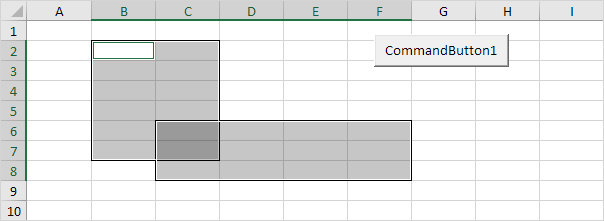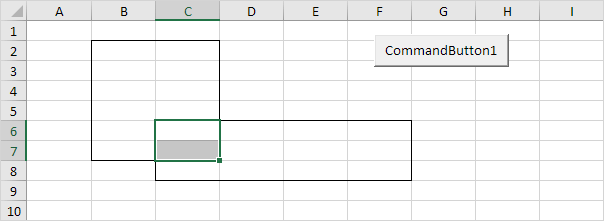# Union and Intersect

The Union method in Excel VBA returns a Range object that represents the union of two or more ranges (borders below for illustration only).

Code line:

Union(Range("B2:C7"), Range("C6:F8")).Select

Result:Note: the Union method doesn't return the mathematical union (cell C6 and cell C7 are included twice).

The Intersect method in Excel VBA returns a Range object that represents the intersection of two or more ranges (borders below for illustration only).

Code line:

Intersect(Range("B2:C7"), Range("C6:F8")).Select

Result:Go to Next Chapter: Variables